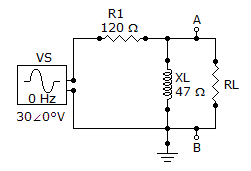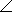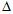# Online Electrical Engineering Test - Electrical Engineering Test 2Loading Test...

Instruction:

• This is a FREE online test. DO NOT pay money to anyone to attend this test.
• Total number of questions : 20.
• Time alloted : 30 minutes.
• Each question carry 1 mark, no negative marks.
• DO NOT refresh the page.
• All the best :-).

1.

When converting 7,000 nA to microamperes, the result is

A.
 0.007 µAB.
 0.7 µAC.
 700 µAD.
 7 µA2.

The quantity 3.3 × 103 is the same as

A.
 330B.
 3,300C.
 33,000D.
 0.00333.

An ohmmeter is an instrument for measuring

A.
 currentB.
 voltageC.
 resistanceD.
 wattage4.

An open circuit is one that has a complete current path.

A.
 TrueB.
 False5.

An electric heater draws 3.5 A from a 110 V source. The resistance of the heating element is approximately

A.
 385B.
 38.5C.
 3.1D.
 316.

If a 24 V and a 6 V battery are series opposing, the total voltage is

A.
 30 VB.
 24 VC.
 18 VD.
 0 V7.

Norton's equivalent current (IN) is an open-circuit current between two points in a circuit.

A.
 TrueB.
 False8.

The angular position of a phasor represents the angle of the sine wave with respect to a reference.

A.
 TrueB.
 False9.

A 0.01F capacitor equals how many picofarads?

A.
 100 pFB.
 1,000 pFC.
 10,000 pFD.
 100,000 pF10.

Inductance is the property of an inductor that produces an opposition to any change in current.

A.
 TrueB.
 False11.

An ac circuit consists of a resistor and a capacitor. To increase the phase angle above 45°, the following condition must exist:

A.
 R = XCB.
 R > XCC.
 R < XCD.
 R = 5XC12.

An imaginary number is one that exists only in the mind of the mathematician.

A.
 TrueB.
 False13.

Referring to Problem 23, the true power is

A.
 68.6 mWB.
 686 mWC.
 564 mWD.
 121.8 mW14.

In a certain series resonant circuit, VC = 125 V, VL = 125 V, and VR = 40 V. The value of the source voltage is

A.
 125 VB.
 250 VC.
 290 VD.
 40 V15.

In applying the superposition theorem,

A.
 the sources are considered one at a time with all others replaced by their internal impedanceB.
 all sources are considered independentlyC.
 all sources are considered simultaneouslyD.
 the sources are considered one at a time with all others replaced by their internal resistance16.

Referring to the given circuit, find ZTH for the part of the circuit that is external to RL.A.
 12921.4°B.
 43.768.6°C.
 43.721.4°D.
 12.968.6°17.

In order to get maximum power transfer from a capacitive source, the load must

A.
 have a capacitive reactance equal to circuit resistanceB.
 have an impedance that is the complex conjugate of the source impedanceC.
 be as capacitive as it is inductiveD.
 none of the above18.

In an electric circuit, the reaction of a circuit to a given pulse input is known as the pulse response.

A.
 TrueB.
 False19.

A three-phase-connected generator is driving a balanced load such that each phase current is 12 A in magnitude. When I θa = 1230° A, what are the polar expressions for the other phase currents?

A.
 I θb = 12150° A, I θc = 12–90° AB.
 I θb = 12120° A, I θc = 1230° AC.
 I θb = 1230° A, I θc = 12120° AD.
 I θb = 1290° A, I θc = 1290° A20.

In a-connected generator, all of the phase voltages are

A.
 zeroB.
 equal in magnitudeC.
 one-third of totalD.
 one-sixth of total